# Qubits World Instructions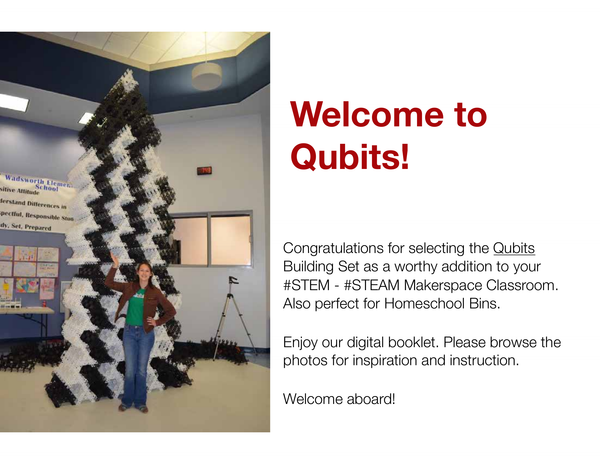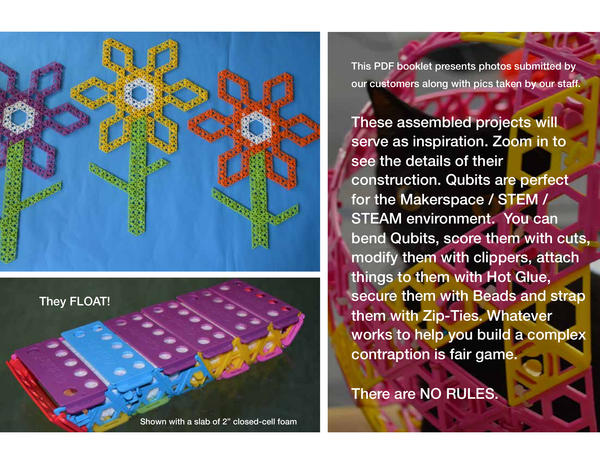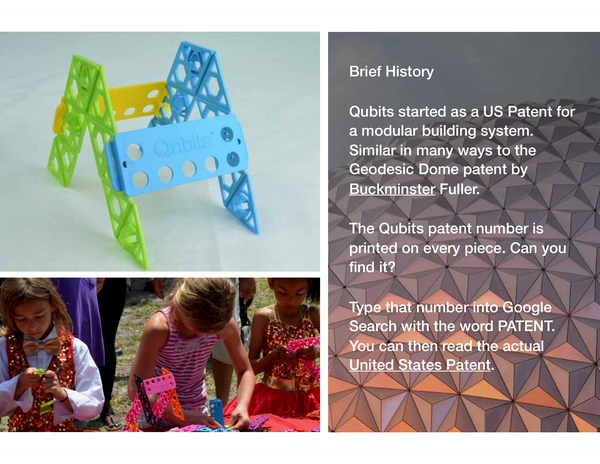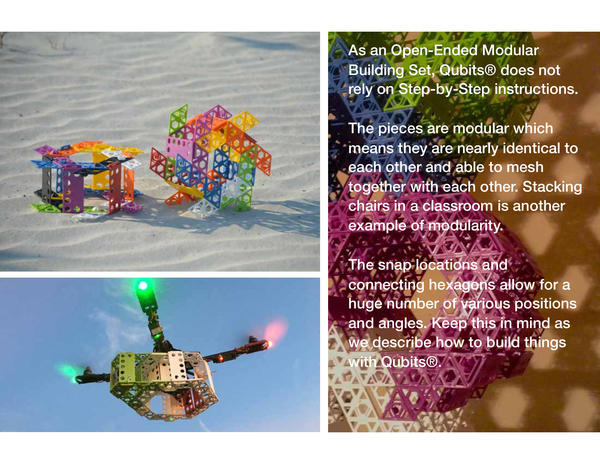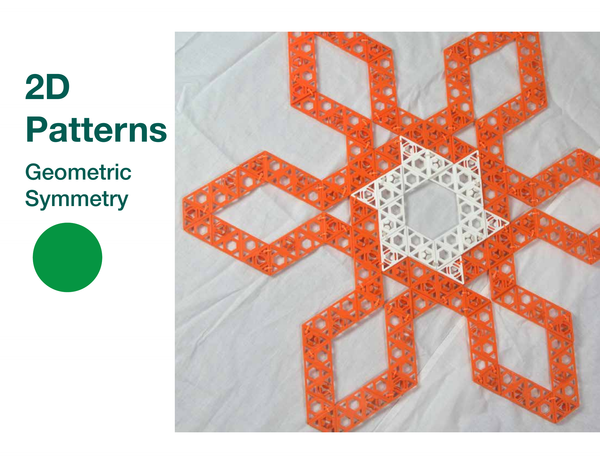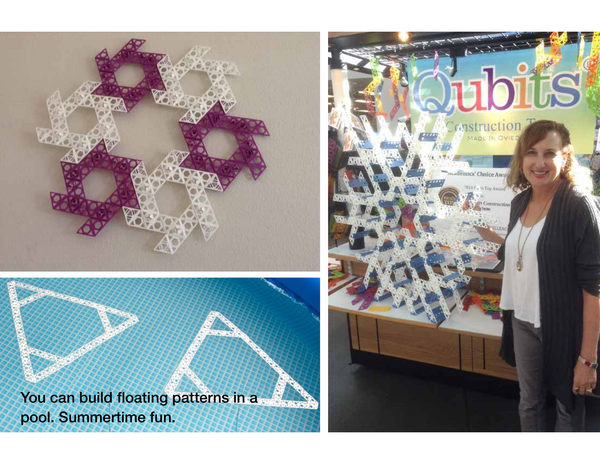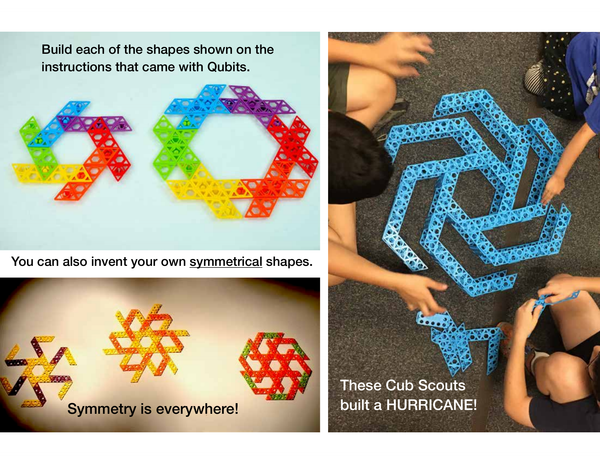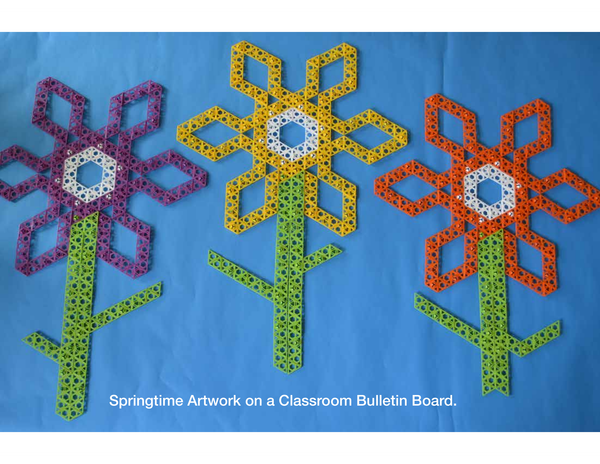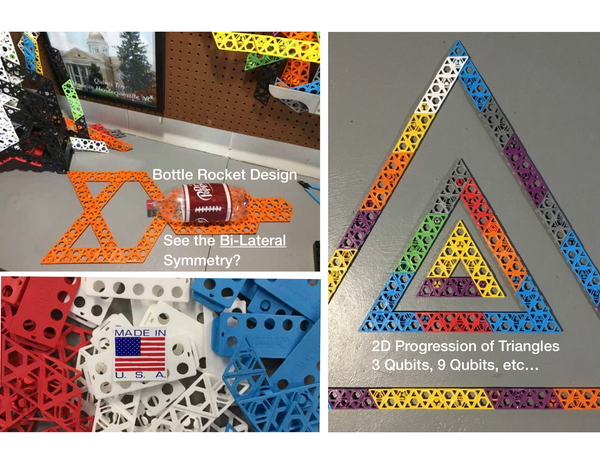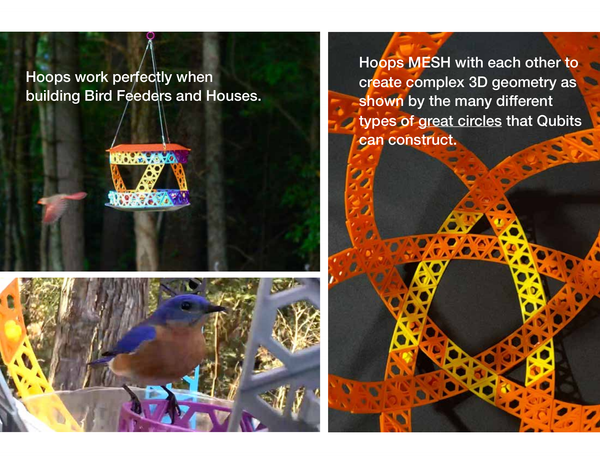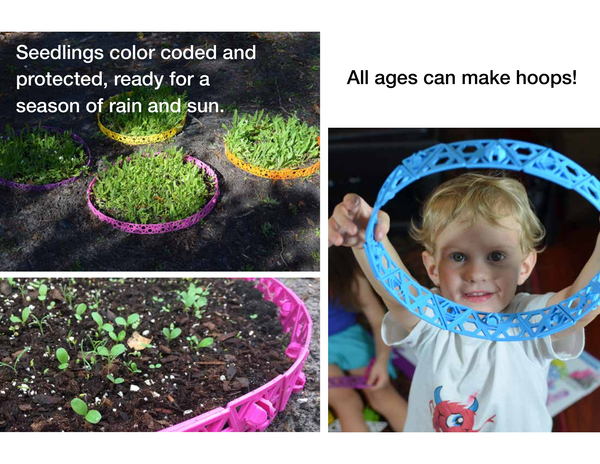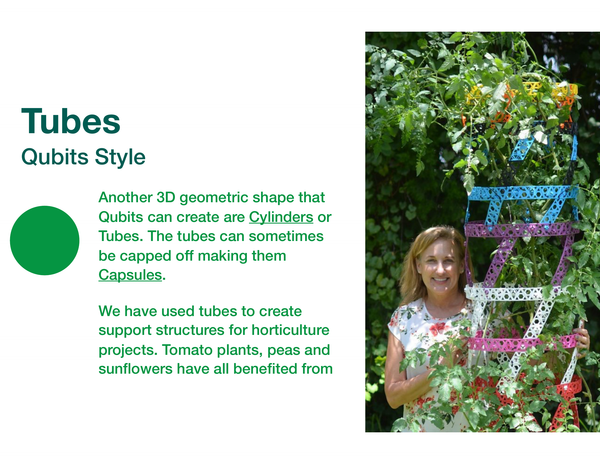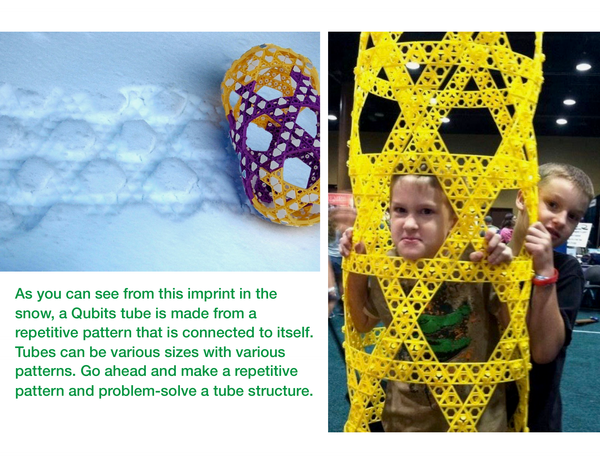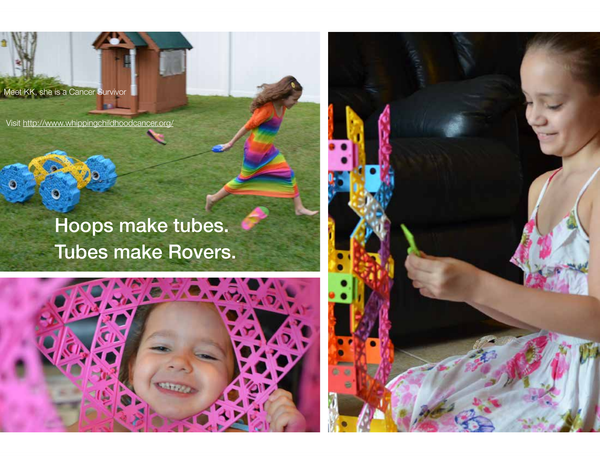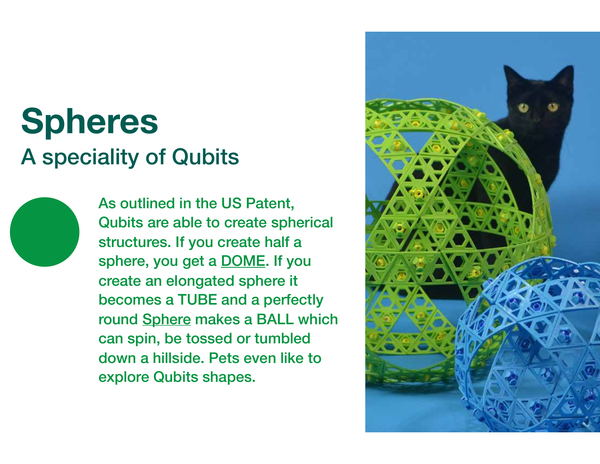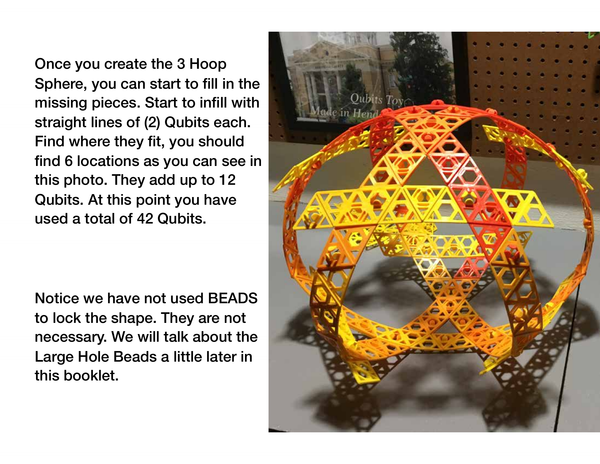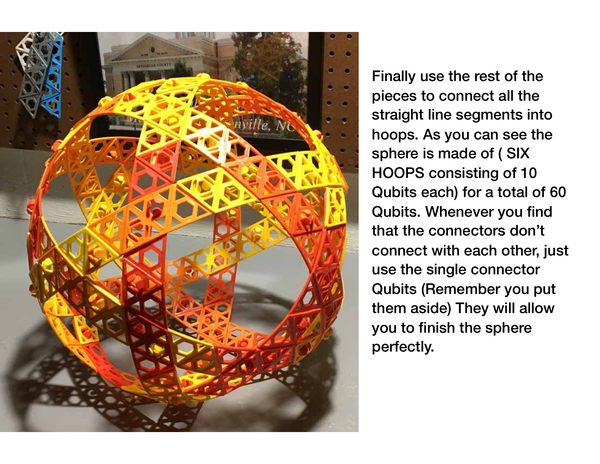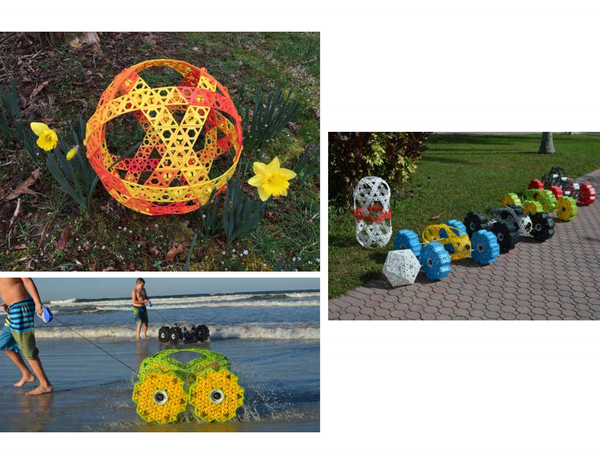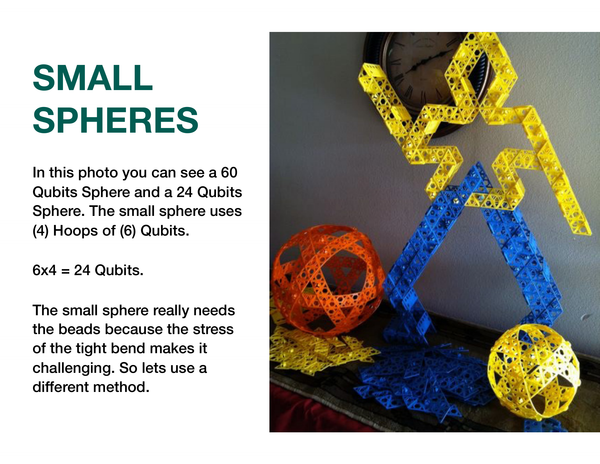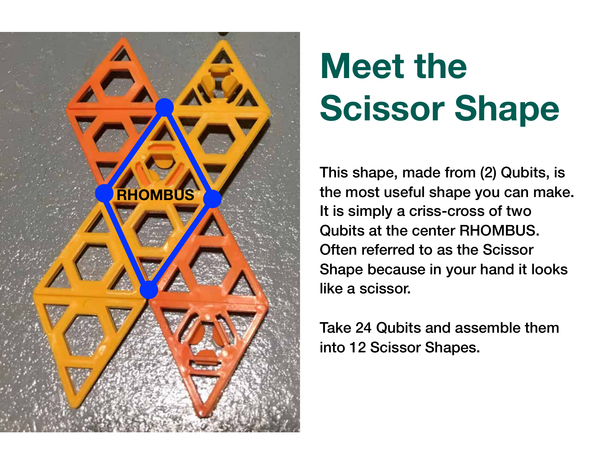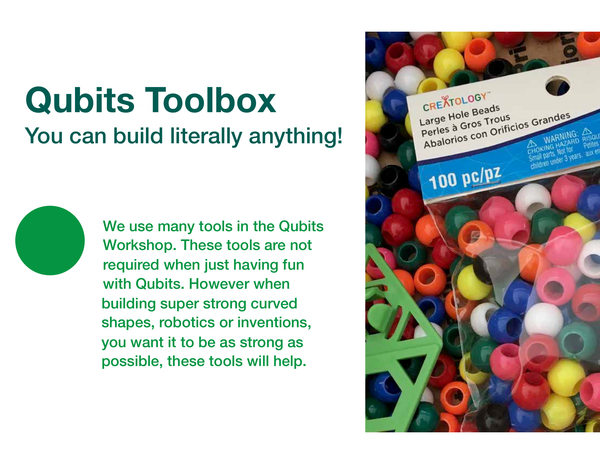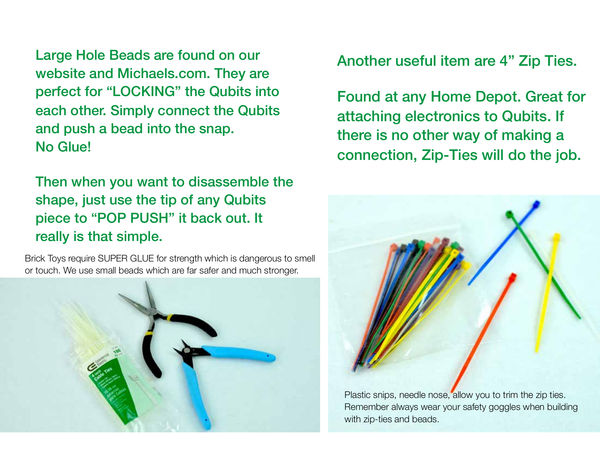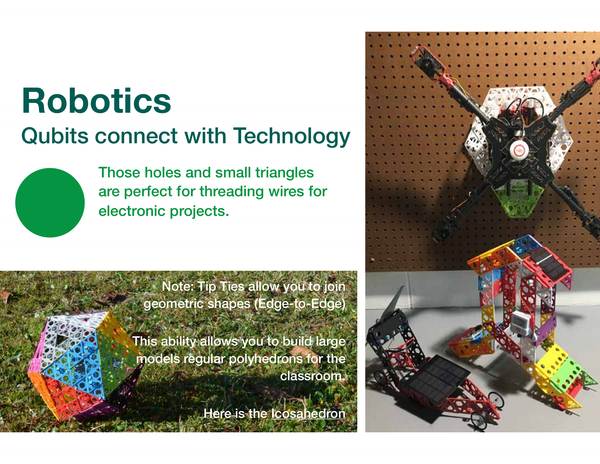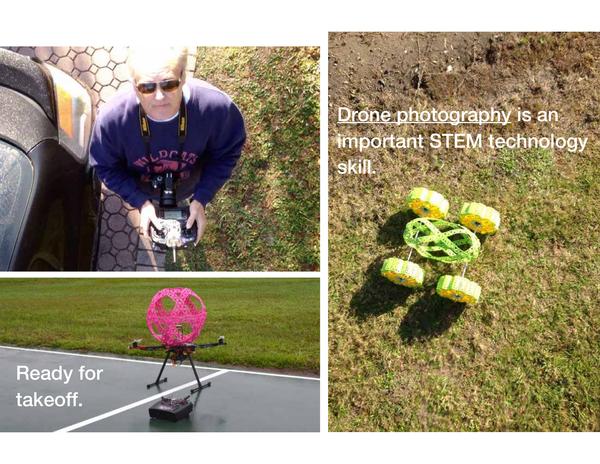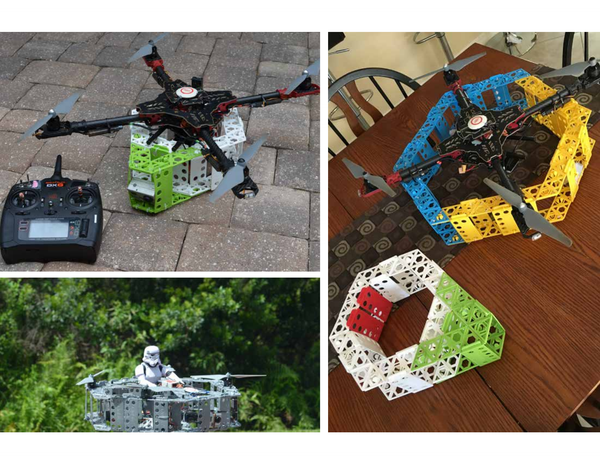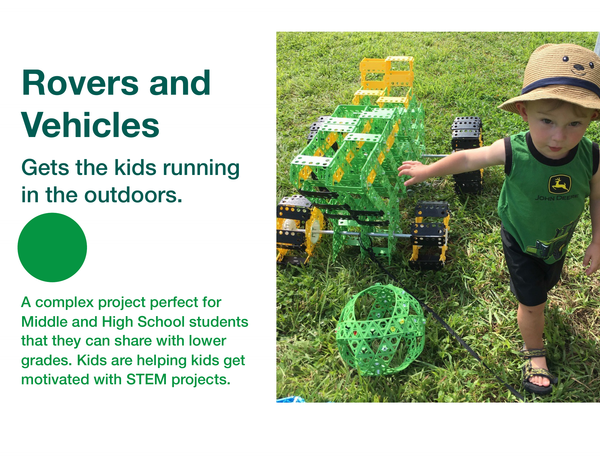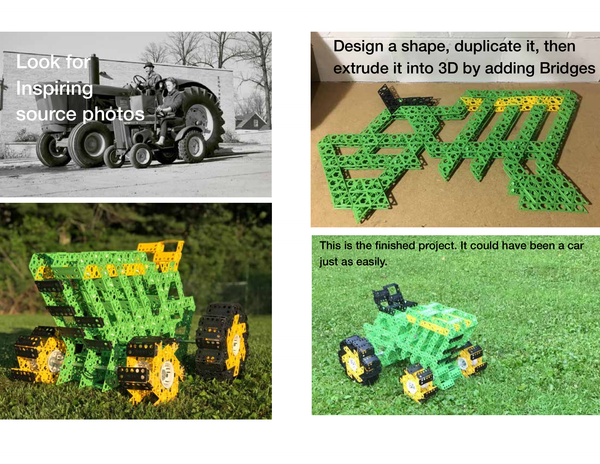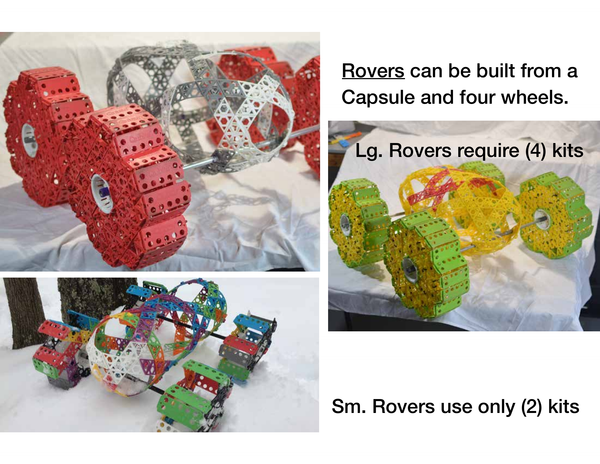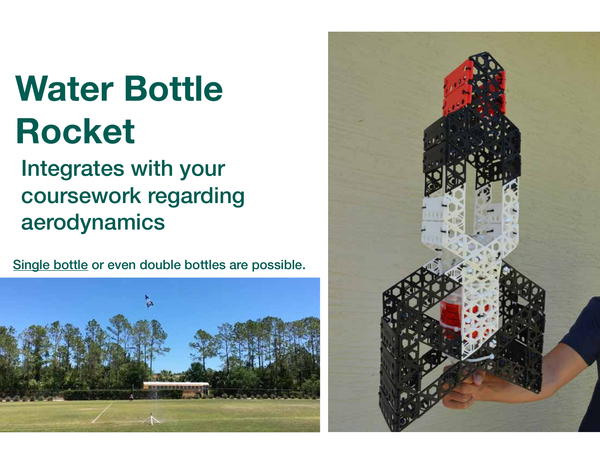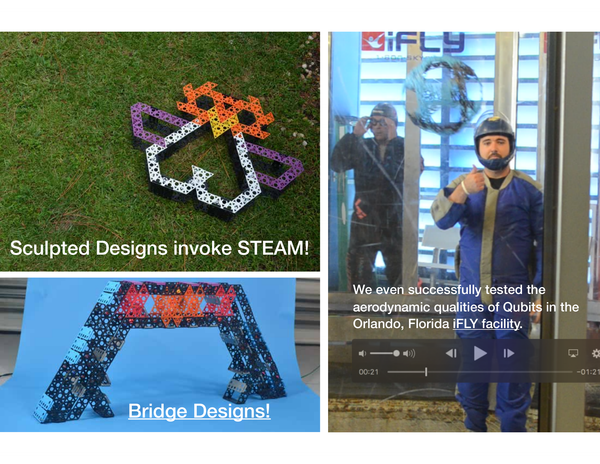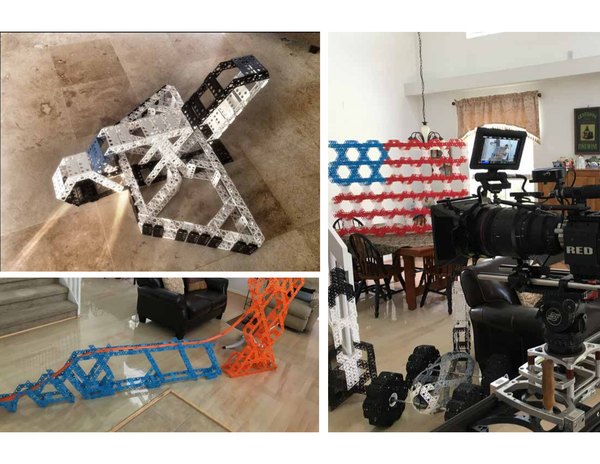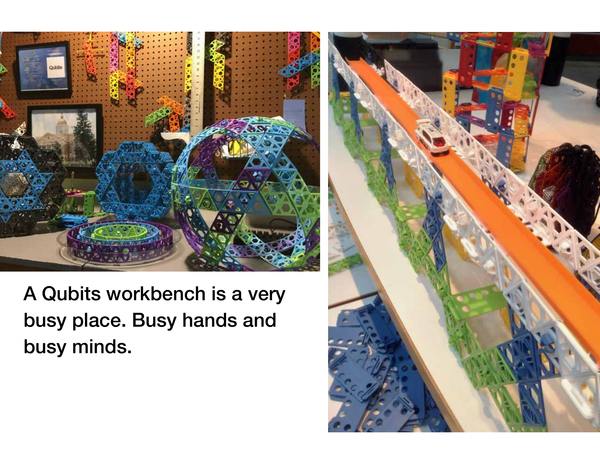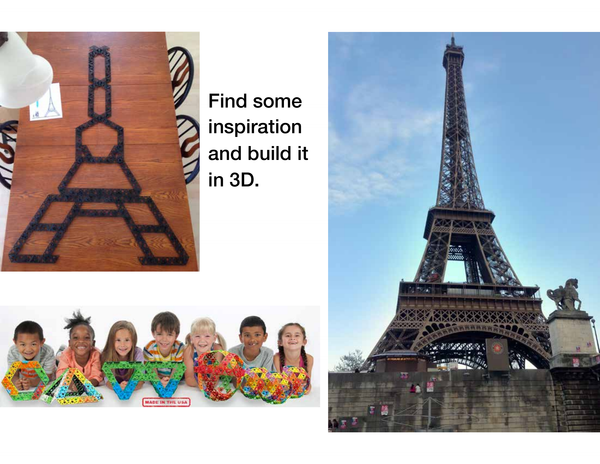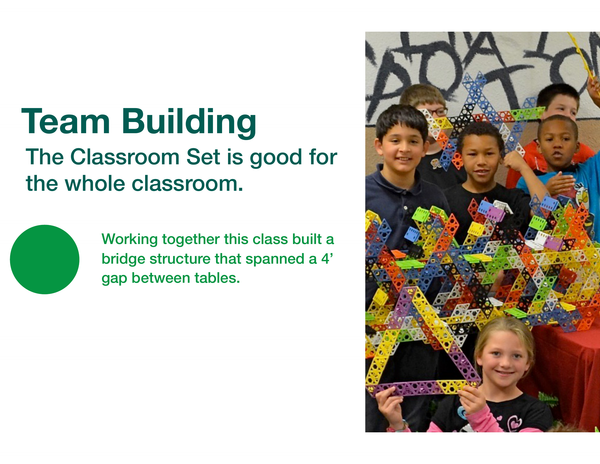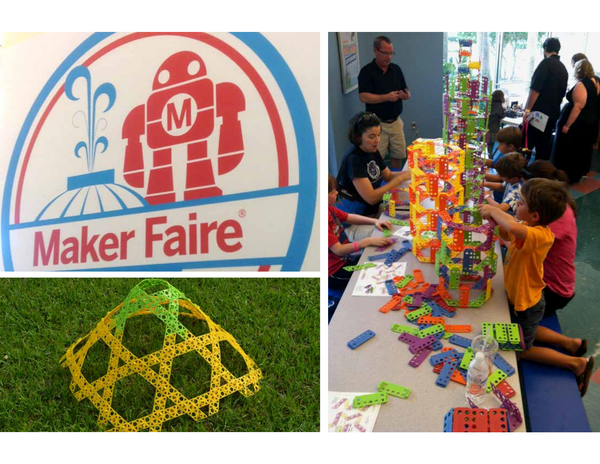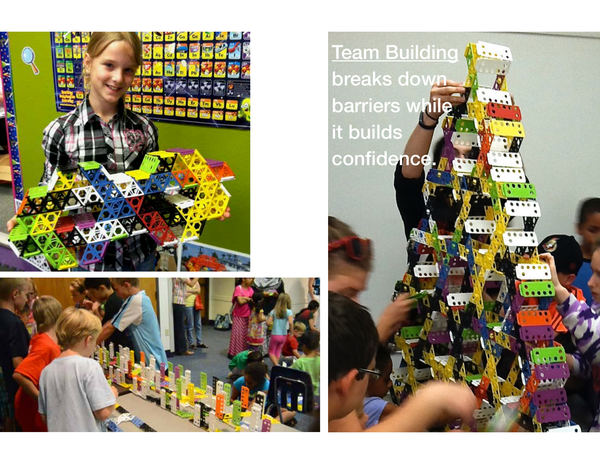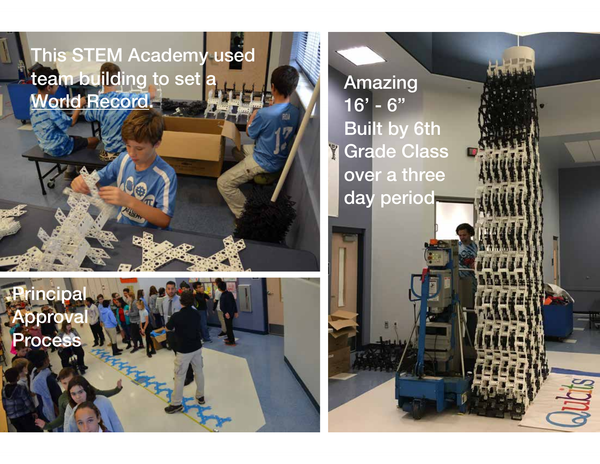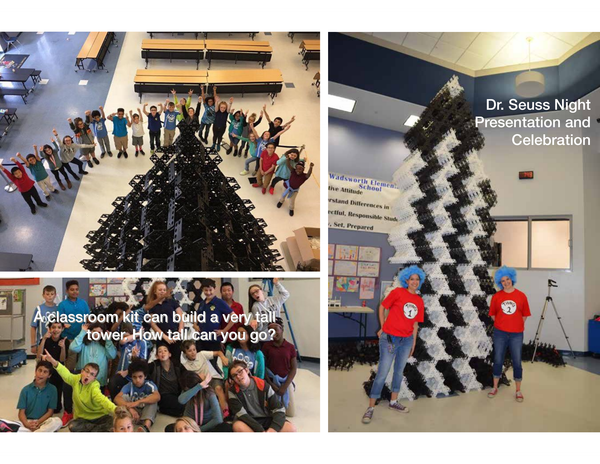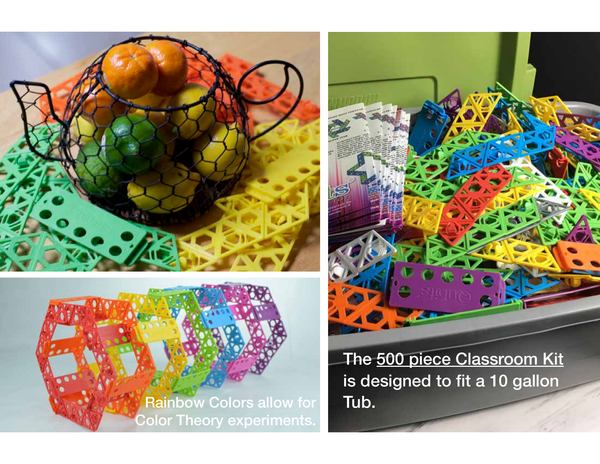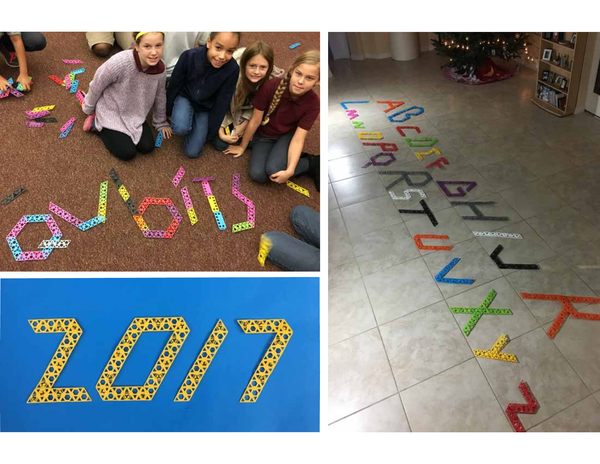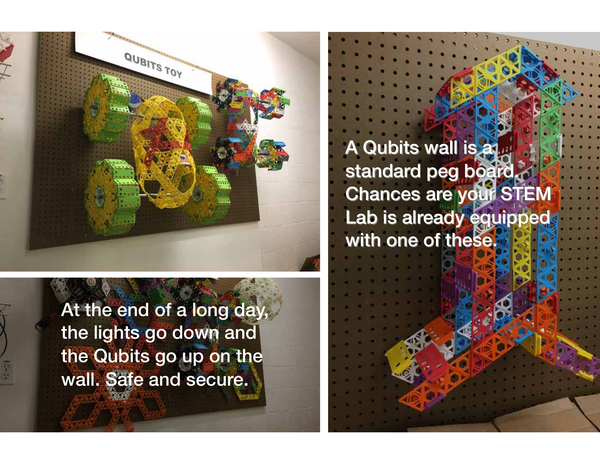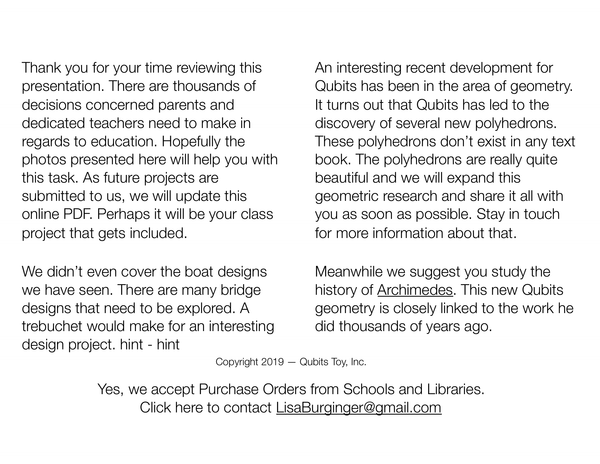### CCSS

The Common Core State Standards (CCSS) are a set of educational benchmarks in the United States that detail what K-12 students should know in English language arts and mathematics at the end of each grade. While the Qubits Toy is primarily a tool for teaching and learning in STEM (Science, Technology, Engineering, and Mathematics) areas, it can potentially align with various Common Core standards, particularly in mathematics and practices of scientific investigation and engineering design.

Here are some Common Core items that could potentially be satisfied through educational activities with Qubits Toy:

1. Mathematics Standards:

• Counting & Cardinality (K): Understanding numbers, counting, and the relationship between numbers and quantities.
• Operations & Algebraic Thinking (Grades 1-5): Represent and solve problems involving addition, subtraction, multiplication, and division.
• Number & Operations in Base Ten (Grades K-5): Use place value understanding and properties of operations to perform multi-digit arithmetic.
• Measurement & Data (K-5): Represent and interpret data; understand concepts of angle and measure angles.
• Geometry (K-8): Analyze, compare, create, and compose shapes. Understand the properties of shapes and the structure of space.
2. Mathematical Practice Standards:

• Make sense of problems and persevere in solving them: Students can use Qubits to model real-world problems and think through solutions.
• Reason abstractly and quantitatively: Working with Qubits requires understanding the abstract properties of shapes and how they can be combined.
• Construct viable arguments and critique the reasoning of others: Students can explain their Qubits constructions and the mathematical reasoning behind them.
• Model with mathematics: Qubits can be used to model geometric concepts and relationships.
• Use appropriate tools strategically: Qubits are a physical tool that can be used to explore mathematical concepts.
• Attend to precision: Building with Qubits requires precision to ensure structures are stable and symmetrical.
• Look for and make use of structure: Understanding the structure of Qubits pieces can lead to more complex and efficient designs.
• Look for and express regularity in repeated reasoning: Students can recognize patterns in their constructions and use that knowledge to solve problems.
3. Science & Engineering Practices:

• Asking questions and defining problems: What can be built with Qubits? How can certain structures be created?
• Developing and using models: Use Qubits to build models of molecules, cells, or architectural structures.
• Planning and carrying out investigations: Experiment with different Qubits configurations to test stability and function.
• Analyzing and interpreting data: Compare the strength and functionality of different Qubits constructions.
• Using mathematics and computational thinking: Apply geometric and mathematical concepts to Qubits designs.
• Constructing explanations and designing solutions: Use Qubits to solve problems or demonstrate scientific principles.
• Engaging in argument from evidence: Discuss why certain Qubits structures work or don't work.
• Obtaining, evaluating, and communicating information: Share findings and designs made with Qubits.

For a more detailed mapping of Qubits Toy to the Common Core standards, it would be necessary to look at specific activities and lessons that are designed using Qubits. Teachers and educators often create lesson plans that outline how a toy or tool like Qubits can be used to meet specific educational standards.